# Sturm-Liouville equation

(diff) ← Older revision | Latest revision (diff) | Newer revision → (diff)

An ordinary differential equation of the second orderwherevaries in a given finite or infinite interval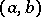,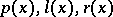are given coefficients,is a complex parameter, andis the sought solution. Ifare positive,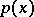has a first derivative and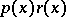has a second derivative, then by the Liouville substitution (see ) this equation may be reduced to the standard form(1)

It is assumed that the complex functionis measurable onand summable on each of the subintervals in it. At the same time one also considers the non-homogeneous equation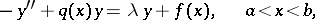(2)

whereis a given function.

Ifis measurable onand summable on each of the subintervals in it, then for all complex numbersand any interior point, equation (2) has onone and only one solutionsatisfying the conditions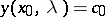,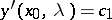. For any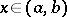the functionis an entire analytic function of. Asone can take one of the end-points of(if this end-point is regular, cf. Sturm–Liouville operator).

Let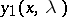andbe two arbitrary solutions of (1). Their Wronskian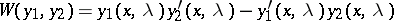is independent ofand vanishes if and only if these solutions are linearly dependent. The general solution of (2) is of the formwhere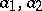are arbitrary constants and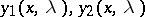are linearly independent solutions of (1).

The following fundamental theorem of Sturm (see ) is true: Let two equations(3)(4)

be given. If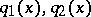are real and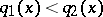on the entire interval, then between any two zeros of any non-trivial solution of the first equation there is at least one zero of each solution of the second equation.

The following theorem is known as the comparison theorem (see ): Let the left-hand end-point ofbe finite, let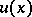be a solution of (3) satisfying the conditions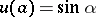,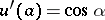, and let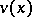be a solution of (4) with the same conditions; let, moreover,on the whole interval. Then, ifhaszeros on,will have at leastzeros and the-th zero ofwill be less than the-th zero of.

One of the important properties of (1) is the existence of so-called operator transforms with a simple structure. Operator transforms arose from general algebraic considerations related to the theory of generalized shift operators (change of the basis).

There are the following types of operator transforms for equation (1). Letbe the solution of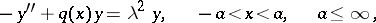(5)

satisfying the conditions(6)

It turns out that this solution has the following representation: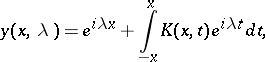where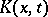is a continuous function independent of; moreover,The integral operator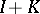defined by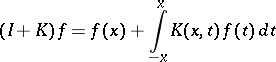is called an operator transform (a transmutation operator), and preserves the conditions at the point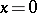. It transforms the function(a solution of the simplest equation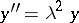with the conditions (6)) into the solution of (5) under the same conditions at the point. Let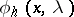and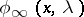be the solutions of (5) satisfying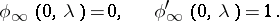These solutions have the representations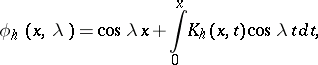whereand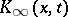are continuous functions.

A new type of operator transforms has been introduced (see ) that preserves the asymptotic behaviour of solutions at infinity; namely, it turned out that for allin the upper half-plane,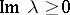, the equation (5), considered on the half-lineunder the conditions, has a solution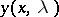that can be represented in the form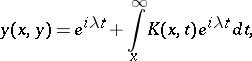whereis a continuous function satisfying the inequality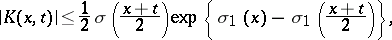in which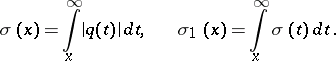Moreover,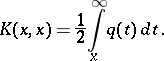How to Cite This Entry:
Sturm-Liouville equation. Encyclopedia of Mathematics. URL: http://encyclopediaofmath.org/index.php?title=Sturm-Liouville_equation&oldid=16467
This article was adapted from an original article by G.Sh. GuseinovB.M. Levitan (originator), which appeared in Encyclopedia of Mathematics - ISBN 1402006098. See original article